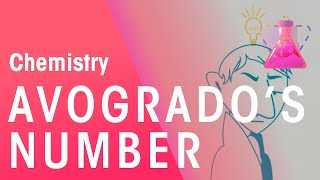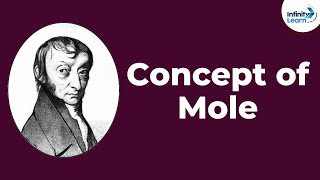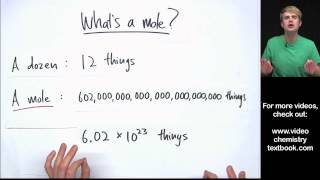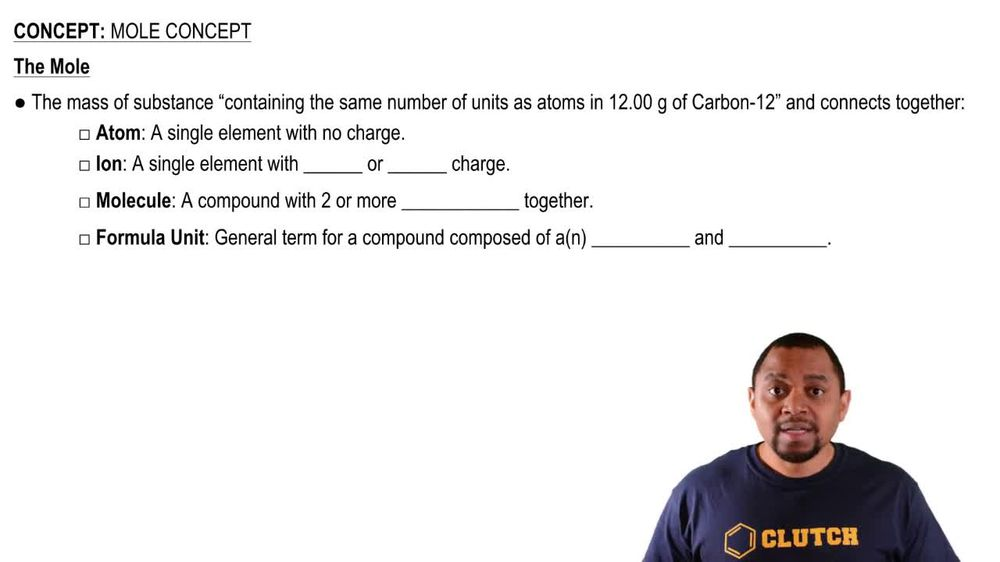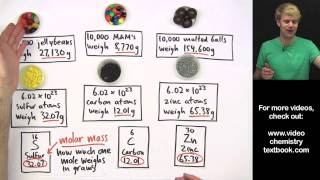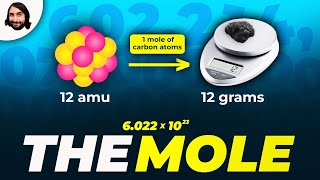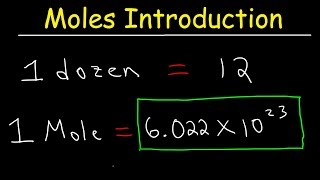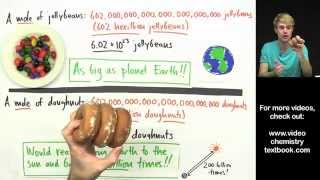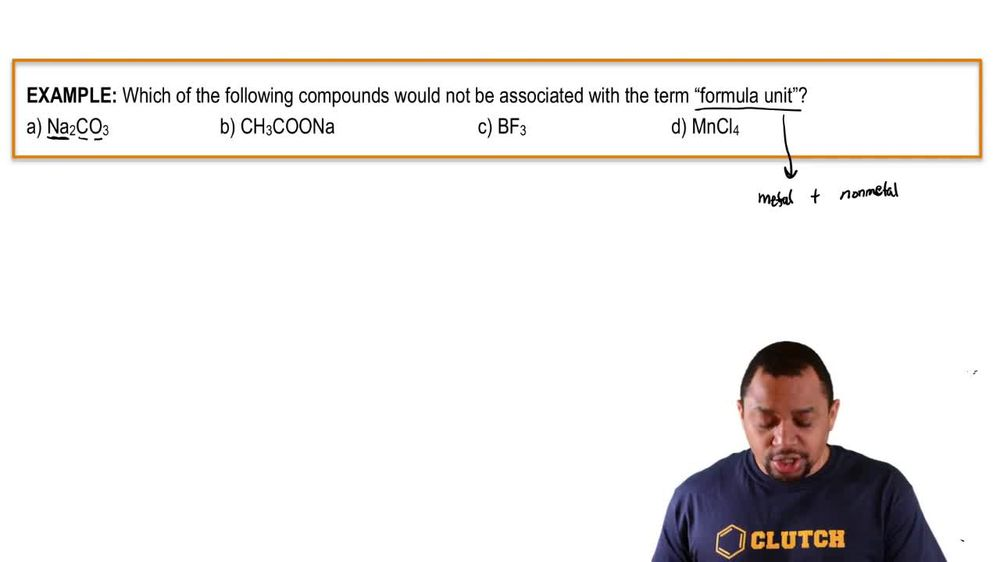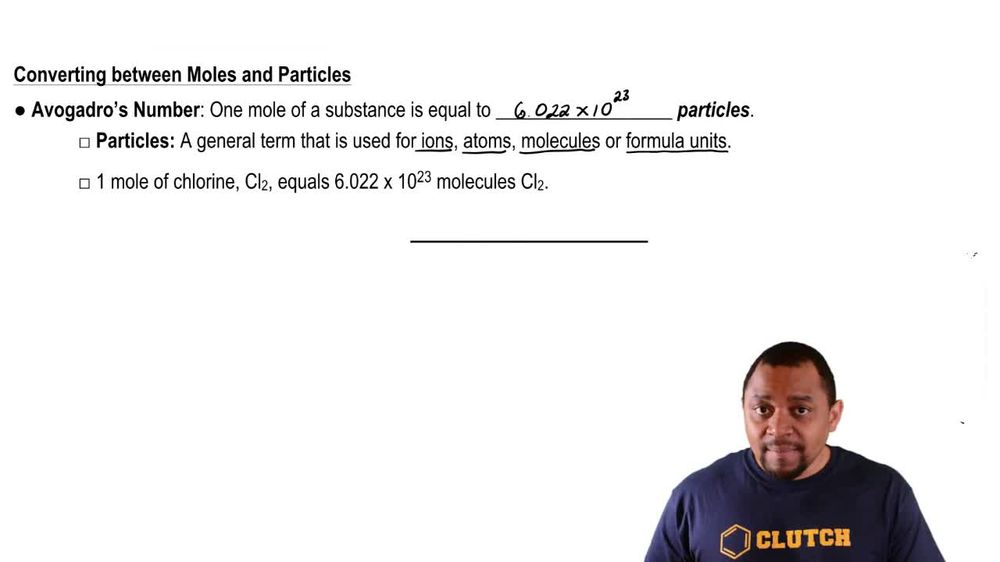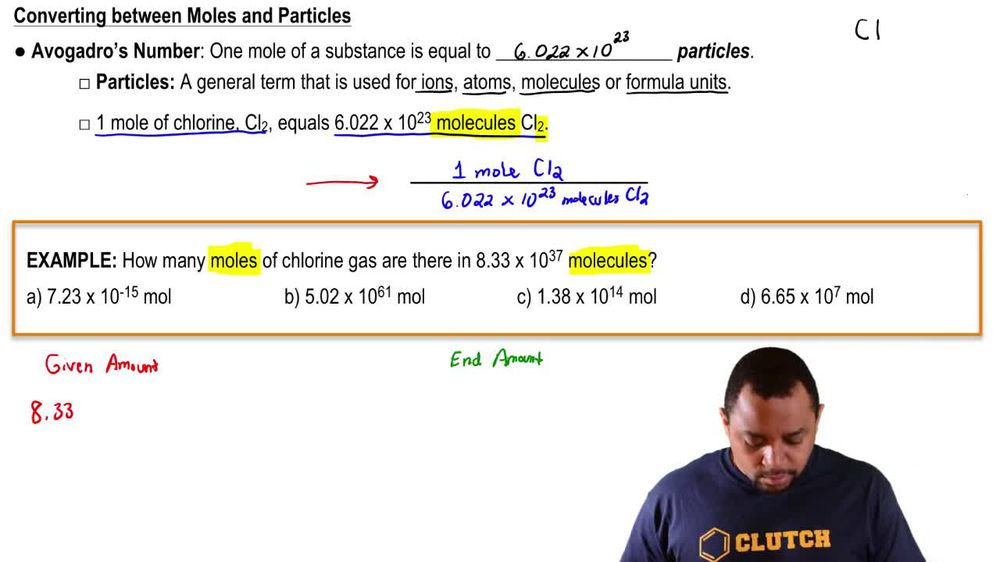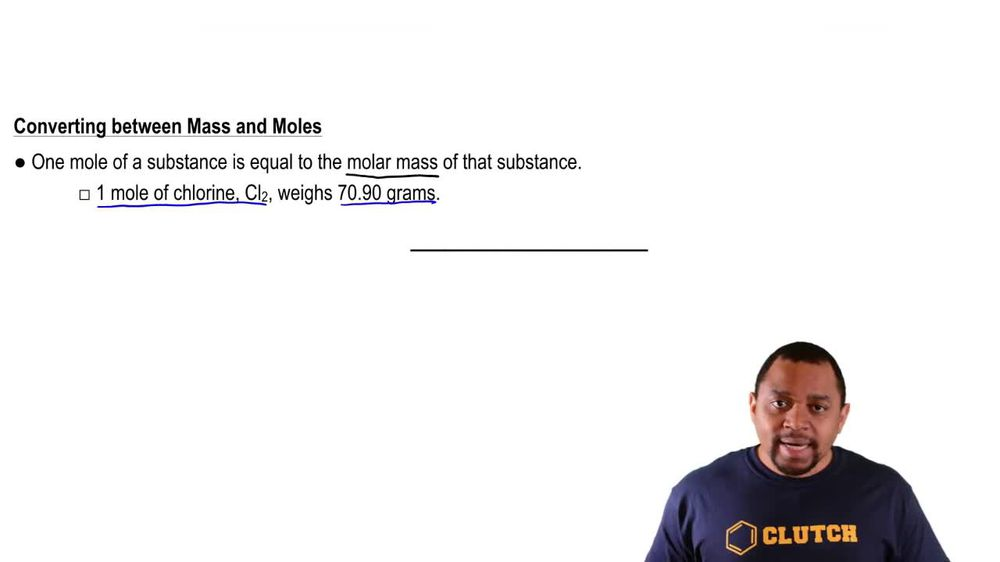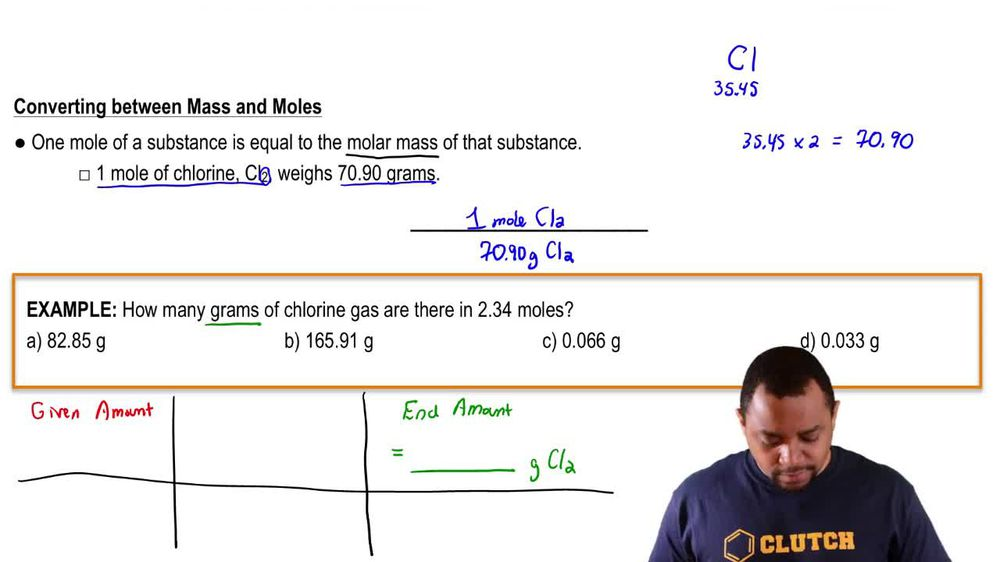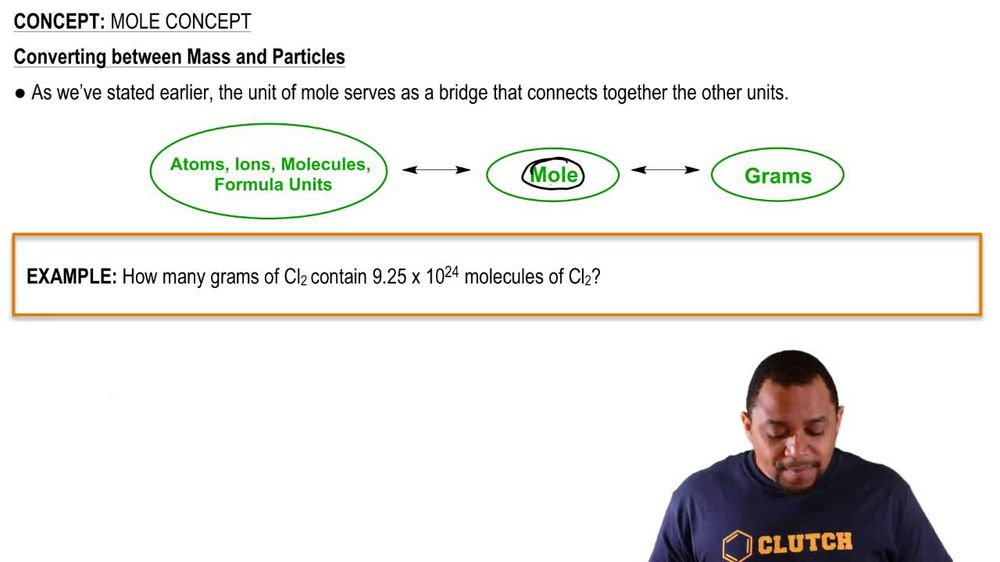Start typing, then use the up and down arrows to select an option from the list.
1. 2. Atoms & Elements2. Mole Concept
Problem

# The molecular formula of saccharin, an artificial sweetener, is C7H5NO3S. (b) How many moles of sachharin are in 2.00 mg of this substance?

Relevant Solution1m
Play a video:
Hi everyone here we have a question telling us that an alternative sweetener stevia has a molecular formula of C. 54 age 70 oh 23. And our goal is to calculate the malls of stevia in 5.0 mg of this substance. So first we need to calculate the molar mass of stevia. So we have carbon and we have 44 of them. And if we look on the periodic table we see that smaller masses 12.01, which equals 528.44. And then we have hydrogen. We have 70 of them times 1. equals 70.7. And then we have oxygen. We have 23 of them And it's more masses 16. That equals 368. For a total of 967. g per mole. So we have 5.00 mg of stevia. And we first need to change that into g. So we're gonna multiply by 10 to the negative 3rd g Divided by one mg times one more Divided by its Molar mass 967. g. And our milligrams are going to cancel out our grams are gonna cancel out, leading us with 5.17 times 10 to the negative six g. And that is our final answer. Thank you for watching. Bye